Question

Use the amounts of water and 0.600 M HCl solution found in Table 3 of your worksheets to calculate the concentrations of the eight dilute HCl solutions you will use in the lab. Do this for Flask 3 and enter your answer in the box below.

This is the information from table 3 flask #3:

volume of water (mL): 25

Volume of HCl (mL): 10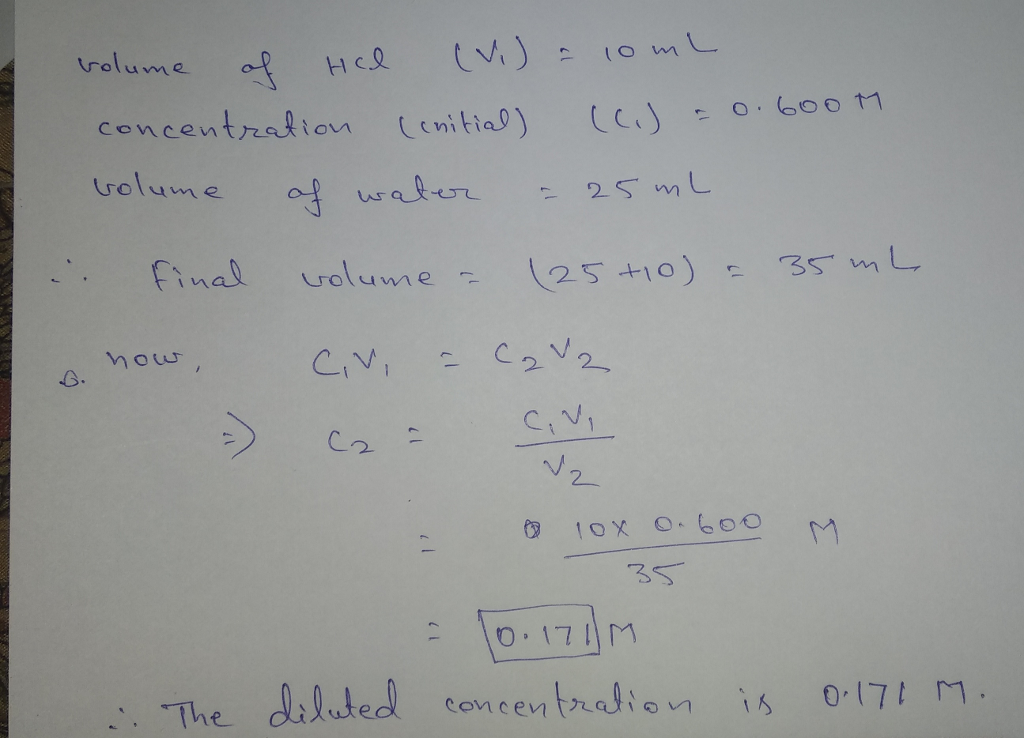#### Earn Coins

Coins can be redeemed for fabulous gifts.

Similar Homework Help Questions
• ### Calculate the concentration of the standard HCl solution you prepared. Determine this concentration for each trial...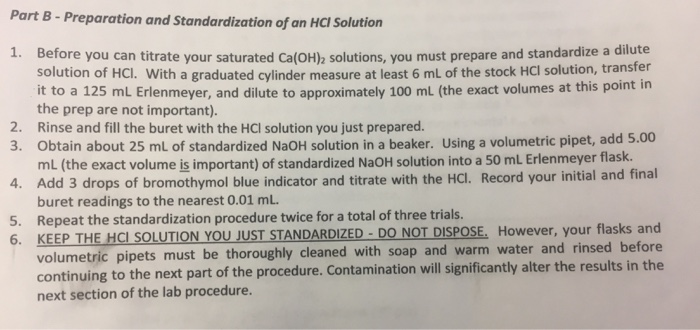Calculate the concentration of the standard HCl solution you prepared. Determine this concentration for each trial and the average and standard deviation for all three trials. Part B-Preparation and standardization of an HCl Solution 1. Before you can titrate your saturated Ca(oH)a solutions, you must prepare and standardize a dilute solution of Hcl. With a graduated cylinder measure at least 6 mL of the stock Hcl lution, transfer it to a 125 mL Erlenmeyer, and dilute to approximately 100 mL...

• ### Enter your answer in the provided box. Calculate the composition of the following solution in mass/volume...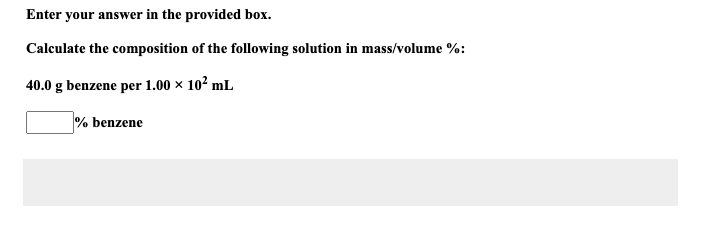Enter your answer in the provided box. Calculate the composition of the following solution in mass/volume %: 40.0 g benzene per 1.00 x 102 mL % benzene Calculate the volume, in L, of water that must be added to dilute 22.9 mL of 10.5 M HCl to 0.0900 M HCl. Enter your answer in the provided box. L water

• ### QUESTIONS 1. Determine the concentration of the HCL solution from the data for the standardization of...

QUESTIONS 1. Determine the concentration of the HCL solution from the data for the standardization of the HCL with the Na2CO3 2. using your data, calculate the moles and mass of acetylsalicylic acid in each tablet (molar mass=180.16g/mol) 3. Using your data, calculate the % by mass of aspirin in the tablet DATA: 1. Make two solutions; Add 1 tablet of aspirin to an erlenmeyer flask 2. using a 50mL pipette, add 50.0mL of NaOH to the tablets in the...

• ### Use a volumetric pipet to deliver 2 mL of 4 M sodium hydroxide solution to each...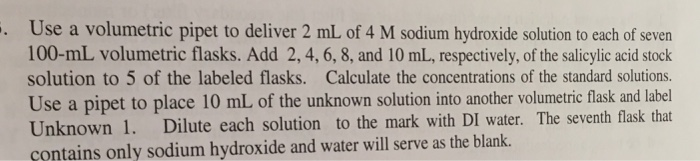Use a volumetric pipet to deliver 2 mL of 4 M sodium hydroxide solution to each of seven 100-mL volumetric flasks. Add 2,4, 6, 8, and 10 mL, respectively, of the salicylic acid stock solution to 5 of the labeled flasks. Calculate the concentrations of the standard solutions. Use a pipet to place 10 mL of the unknown solution into another volumetric flask and label Unknown 1. Dilute each solution to the mark with DI water. The seventh flask that...

• ### Table A. Preparation of Standard solutions of FeSCN2+ 1.0 M HNO3 0.002 M 0.200 M Solution...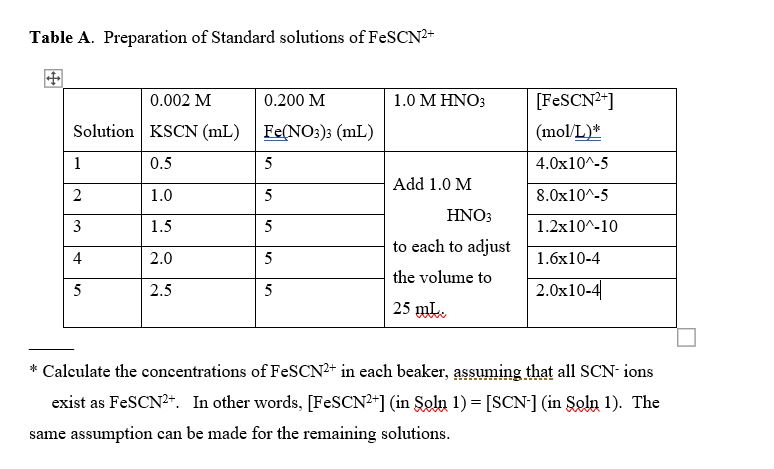Table A. Preparation of Standard solutions of FeSCN2+ 1.0 M HNO3 0.002 M 0.200 M Solution KSCN (mL) Fe(NO3)3 (mL) 0.5 5 [FeSCN2+] (mol/L)* 1 4.0x10^-5 Add 1.0 M 2 1.0 5 8.0x10^-5 HNO3 3 1.5 5 1.2x10^-10 4 2.0 5 1.6x10-4 to each to adjust the volume to 25 mL. 5 2.5 5 2.0x10-4 * Calculate the concentrations of FeSCN2+ in each beaker, assuming that all SCN-ions exist as FeSCN2+. In other words, [FeSCN2+] (in Soln 1) = [SCN-]...

• ### Calculate the initial concentration of iron(III) in solution #1, #2, #3. Beaker (mL) Table 1. Reagent...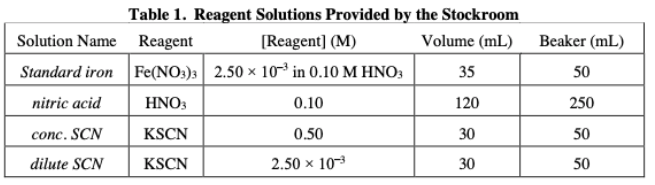Calculate the initial concentration of iron(III) in solution #1, #2, #3. Beaker (mL) Table 1. Reagent Solutions Provided by the Stockroom Solution Name Reagent [Reagent] (M) Volume (mL) Standard iron Fe(NO3)3 2.50 x 10 in 0.10 M HNO3 nitric acid HNO3 0.10 120 35 50 250 conc. SCN KSCN 0.50 30 50 dilute SCN KSCN 2.50 x 10-3 30 50 Worksheet 1 #1 5.00 #2 10.00 #3 15.00 Dilute iron (mL) [Fe**] (M) [Fe(SCN)?*] (M) %T 480 58.9 35.8 21.5...

• ### Q3.1 3 Points A 12.0 M stock solution of HCl(aq) is diluted with water to give...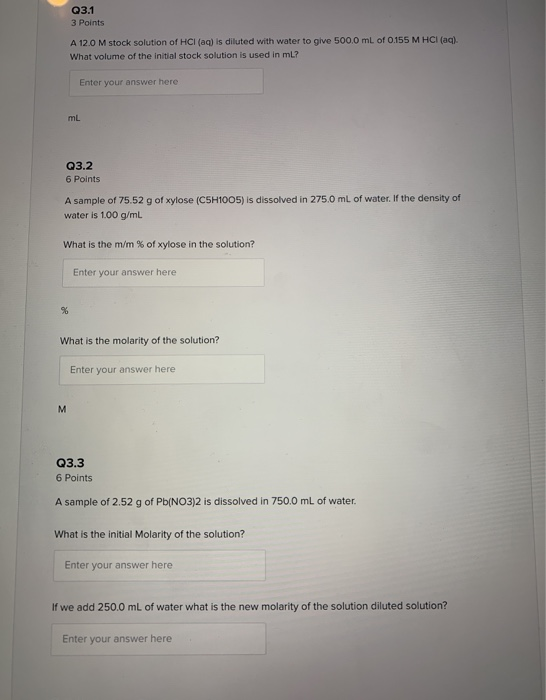Q3.1 3 Points A 12.0 M stock solution of HCl(aq) is diluted with water to give 500.0 ml of 0.155 M HCl(aq). What volume of the initial stock solution is used in ml? Enter your answer here mL Q3.2 6 Points A sample of 75.52 g of xylose (C5H1005) is dissolved in 275.0 mL of water. If the density of water is 1.00 g/ml What is the m/m % of xylose in the solution? Enter your answer here What is...

• ### 6) A stock solution of HCl is 11.50 M, if 15.29 ml of the stock solution...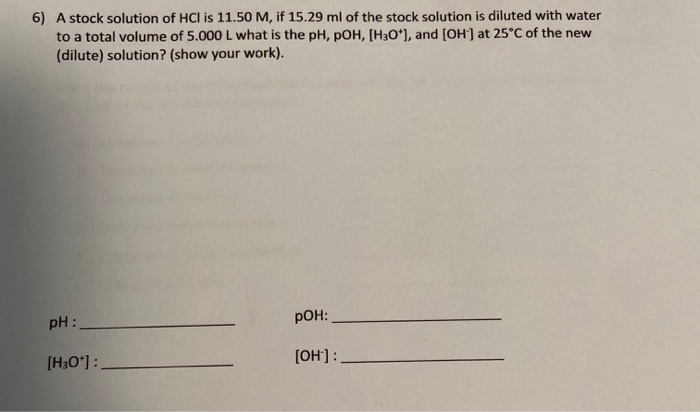6) A stock solution of HCl is 11.50 M, if 15.29 ml of the stock solution is diluted with water to a total volume of 5.000 L what is the pH, POH, [H30*), and (OH') at 25°C of the new (dilute) solution? (show your work). pH : pОН: [H30*): (OH) :

• ### 6) A stock solution of HCl is 11.50 M, if 15.29 ml of the stock solution...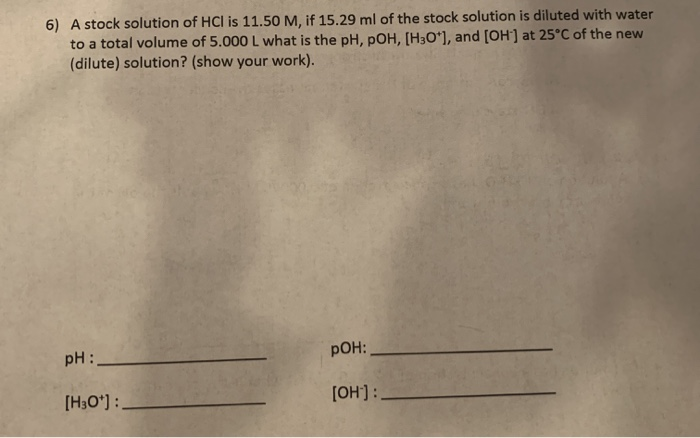6) A stock solution of HCl is 11.50 M, if 15.29 ml of the stock solution is diluted with water to a total volume of 5.000 L what is the pH, POH, [H3O], and (OH) at 25°C of the new (dilute) solution? (show your work). pОН: pH : [OH-] : [H30*) :

• ### 6) A stock solution of HCl is 11.50 M, if 15.29 ml of the stock solution...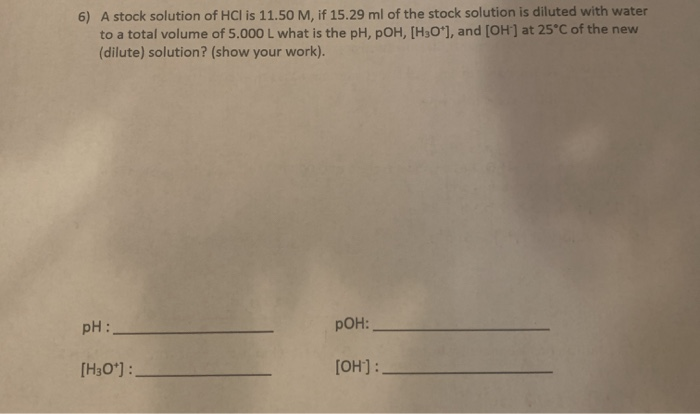6) A stock solution of HCl is 11.50 M, if 15.29 ml of the stock solution is diluted with water to a total volume of 5.000 L what is the pH, POH, [H3O+], and (OH) at 25°C of the new (dilute) solution? (show your work). pH : pОН: [H30*) : [OH) :